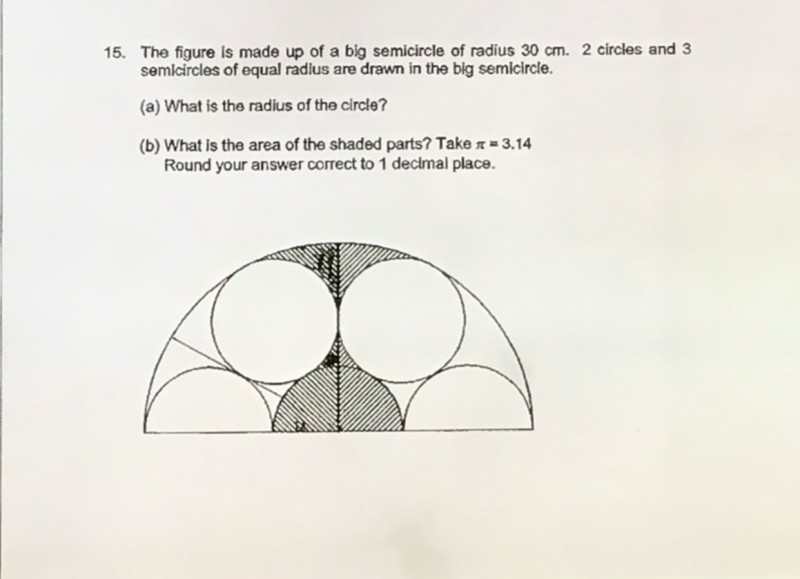# QuestionRadius of big semi circle R= 30cm

Radius of small circle = r

3r = 30

(a)  r = 10cm

(b) Area of big semi circle = (πR2 )/2 = (3.14 x 30 x 30) / 2 = 1413

Area of 1 small semi circles = (πr2 )/2 = (3.14 x 10 x 10) / 2 = 157

Area of 7 small semi circles = 7 x  (πr2 )/2 = 7 x  157 = 1099

Area in between the small circles within the big semi-circle, A = 1413-1099 = 314